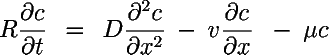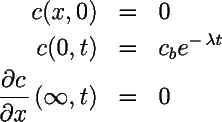# EPA On-line Tools for Site Assessment Calculation

##### Transport and Leaching from a Release of Fresh Fuel

One Dimensional Transport Equation

This calculator generates a breakthrough curve* at a receptor and uses a one-dimensional model to determine the

1. First arrival time for a contaminant (above a specified threshold concentration),
2. Maximum Concentration, and
3. Duration of Contaminantion above the specified threshold

*The breakthrough curve is the time history of concentration at a receptor located a specified distance from the contaminant source.See bottom of page for equations solved.>

Date

mg/L

mg/L

Water Saturation

##### Default Parameters

Percent Mass Fraction

##### Completion of Breakthrough Curve (for Pulse Sources)

One Dimensional Transport EquationR = retardation factor []
D = dispersion constant [L2/T]
v = seepage velocity [L/T]
Î¼ = first order decay constant [1/T]

with the initial and boundary conditionsretardation factor R = 1 + Ï b k d /Î¸

R = retardation factor
Ï b = bulk density = Ï s (1-Î¸)
Ï s = solids density
Î¸ = porosity
k d = (soil) distribution coefficient = f o c K o c
f o c = fraction organic carbon
K o c = organic carbon/water partition coefficient

travel time t t r = x R / q s

t t r = advective travel time*
q s = seepage velocity
R = retardation factor
x = distance